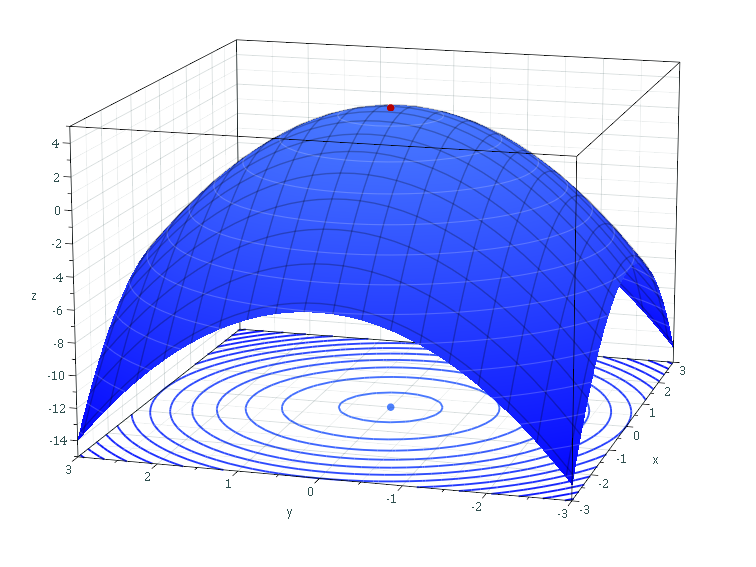# Code Samples from TFCO — TensorFlow Constrained Optimization

Original article was published by Aswin Vijayakumar on Artificial Intelligence on Medium# Code Samples from TFCO — TensorFlow Constrained Optimization

Includes Code Samples from TFCO — TensorFlow Constrained Optimization

The above article models business functions which is equivalent to modelling the conceptual structure of the system. It is always good to model the business process (BPMN) because that is the standardised way of modeling the system. Business Functions model the category of operations of the system routine.

In order to work with Deep Learning Libraries, I have created an article that showcases about TensorFlow Constrained Optimization (TFCO) which works similar to Boxing and Unboxing technique as explained above in the article.

In this example, I have provided a class which assigns the responsibilities to the TensorFlow operations defined in the example. The example here uses Recall constraints which recalls the data objects based on a Hinge Loss. A Recall is a metric that is equivalent to TPR (True Positive Rate). Recalling a data object implies assessing the correctness measure of the object’s existence. The constraint optimization problem is defined within a Class using an Object Oriented Programming fashion. Each constraint of the class is defined in a method as a tensor totally relying on Object Constraint Language (OCL) like syntax. Implying, each method returns a tensor of unit variable for single constraint. The TFCO process takes in one input data point similar to the two data points structure taken by a DEA model. The Data Management Units (DMUs) are similar to weights accepted the TFCO in this model but there is a Characteristic Loss Function as explained below.

Google Research’s TensorFlow Constrained Optimization is a Python Library for performing Machine Learning based Optimizations. In this article, I have taken an example from Recall constraint, which characterises features in the data and minimizes the rejection of objects represented in the data.

# Hinge Loss

Hinge Loss is represented as [0, 1 — y(x)], this implies in the entropy calculation, it does not consider those labels which are true predictions whereas those objects which are classified false are considered for false. A minimization algorithm is performed to reduce the false positives.

The problem is rate minimization problem, where the constraints are defined and the hinge loss is defined.

# Defining the Objective

`# we use hinge loss because we need to capture those that are not classified correctly and minimize that lossdef objective(self):    predictions = self._predictions    if callable(predictions):        predictions = predictions()    return tf.compat.v1.losses.hinge_loss(labels=self._labels,        logits=predictions)`

The objective here is hinge loss with labels representing the true positives and false positives.

# Defining the Constraints

The constraints are defined such that the recall value is at least the lower bound which is mentioned in the problem. In Convex Optimization Case, the constraints are represented as ≥ 0.

`def constraints(self):        # In eager mode, the predictions must be a nullary function  returning a        # Tensor. In graph mode, they could be either such a function, or a Tensor        # itself.        predictions = self._predictions        if callable(predictions):            predictions = predictions()        # Recall that the labels are binary (0 or 1).true_positives = self._labels * tf.cast(predictions > 0, dtype=tf.float32)true_positive_count = tf.reduce_sum(true_positives)recall = true_positive_count / self._positive_count        # The constraint is (recall >= self._recall_lower_bound), which we convert        # to (self._recall_lower_bound - recall <= 0) because        # ConstrainedMinimizationProblems must always provide their constraints in        # the form (tensor <= 0).        #        # The result of this function should be a tensor, with each element being        # a quantity that is constrained to be non-positive. We only have one        # constraint, so we return a one-element tensor.        return self._recall_lower_bound - recall    def proxy_constraints(self):        # In eager mode, the predictions must be a nullary function returning a        # Tensor. In graph mode, they could be either such a function, or a Tensor        # itself.        predictions = self._predictions        if callable(predictions):            predictions = predictions()        # Use 1 - hinge since we're SUBTRACTING recall in the constraint function,        # and we want the proxy constraint function to be convex. Recall that the        # labels are binary (0 or 1).        true_positives = self._labels * tf.minimum(1.0, predictions)        true_positive_count = tf.reduce_sum(true_positives)        recall = true_positive_count / self._positive_count        # Please see the corresponding comment in the constraints property.        return self._recall_lower_bound - recall`

# The Full Example Problem of Recall Constraint

`class ExampleProblem(tfco.ConstrainedMinimizationProblem):    def __init__(self, labels, predictions, recall_lower_bound):        self._labels = labels        self._predictions = predictions        self._recall_lower_bound = recall_lower_bound        # The number of positively-labeled examples.        self._positive_count = tf.reduce_sum(self._labels)@property    def num_constraints(self):        return 1# we use hinge loss because we need to capture those that are not classified correctly and minimize that loss    def objective(self):        pass    def constraints(self):        pass    def proxy_constraints(self):        passproblem = ExampleProblem(    labels=constant_labels,    predictions=predictions,    recall_lower_bound=recall_lower_bound,)`

## Visualization of Constant Input Data for which the Recall is calculated

*Please Note: In this case the problem is originating from the data

`Constrained average hinge loss = 1.185147Constrained recall = 0.845000`

In the article shown above we do not have ever changing data, using existing data we calculate the input data weights in order to predict those samples that produce lowest recall. The predictions from one constrained optimization model is sent to the next model that runs on different loss. This way we can model how those two objects communicate each other.

I’ll leave it up to you guys to decide if Azure ML Studio or AWS Deep Racer can be used to build Machine Learning Models using these ideas.

References